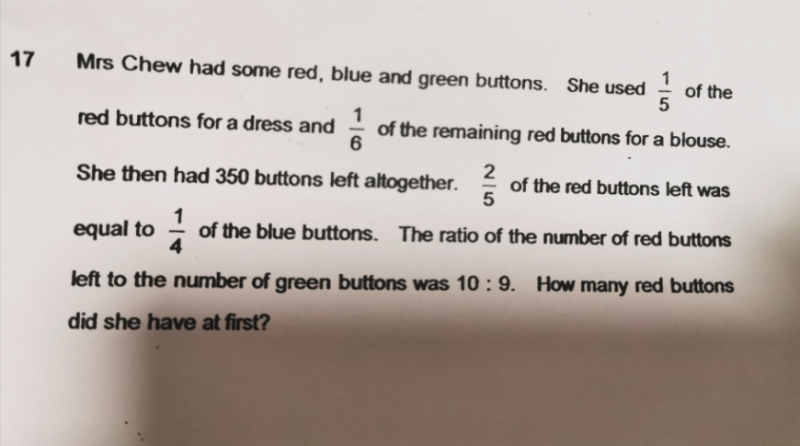# QuestionCan someone pls help ?

Thank you.

Start from the leftovers.

2/5 of red => 1/4 of Blue.
To find the ratio of Red : Blue we make the numerator common.
2/5 of red => 2/8 of Blue

Red : Blue => 5 : 8 => 10 : 16
Red : Blue : Green => 10 : 16 : 9

10u + 16u + 9u = 350
35u = 350
u = 350/35 = 10

Leftover
Red = 10u =100
Blue = 16u = 160
Green = 9u = 90

5/6 of Remaining Red => 100
6/6 of Remaining Red = 100/5 X 6 = 120

4/5 of Red at first => 120
5/5 of Red at first = 120/4 X 5 = 150 ##

0 Replies 0 Likes